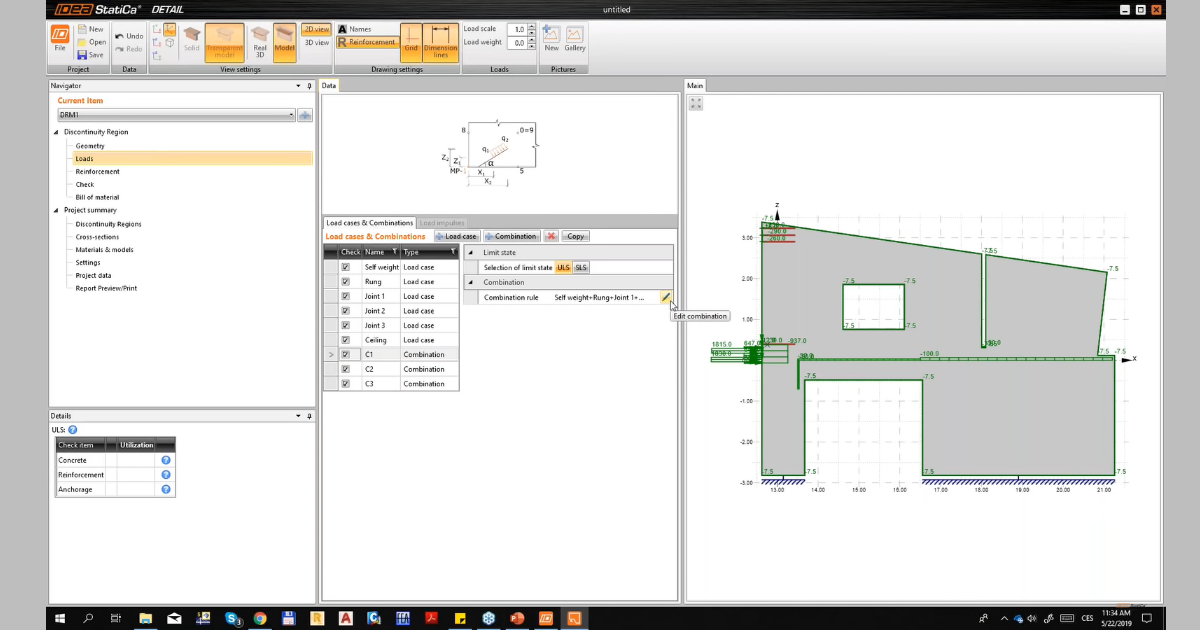# How to input the partial factors for ULS and SLS

Cet article est aussi disponible en :
$$Load cases as self–weight or ceiling are added in the characteristic values. If we input the load cases from the joints, we apply the partial factor of 1 due to the design value extracted from the IDEA StatiCa Connection. Nevertheless in SLS, the situation is not so simple due to the fact that design values cannot be separated from the point of view of permanent and variable loads and divided by appropriate partial factors. The reason is the non-linear analysis in IDEA StatiCa Connection so we cannot use the superposition of the loads. Regarding this effect, we have chosen a partial factor of 0.75, which is coming out from the equation 1/1.35.Watch the part of our webinar dedicated to correct input of the partial factors for ULS and SLS.$$